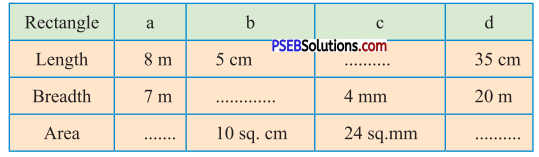# PSEB 5th Class Maths Solutions Chapter 8 Perimeter and Area Ex 8.2

Punjab State Board PSEB 5th Class Maths Book Solutions Chapter 8 Perimeter and Area Ex 8.2 Textbook Exercise Questions and Answers.

## PSEB Solutions for Class 5 Maths Chapter 8 Perimeter and Area Ex 8.2

1. Find the area of following rectangles whose length and breadth are as follows :

Question 1.
9 m and 7 m
Solution:
Length of rectangle = 9 m
Breadth of rectangle = 7 m
Area of rectangle = Length × Breadth
= 9 m × 7 m
= 63 m2Question 2.
85 cm and 76 cm
Solution:
Length of rectangle = 85 cm
Breadth of rectangle = 76 cm
Area of rectangle = Length × Breadth
= 85 cm × 76 cm
= 6460 cm2

Question 3.
23 mm and 18 mm
Solution:
Length of rectangle = 23 mm
Breadth of rectangle = 18 mm
Area of rectangle = Length × Breadth
= 23 mm × 18 mm
= 414 mm2

Question 4.
5 m and 85 cm
Solution:
Length of rectangle
= 5 m
= 5 × 100 cm
= 500 cm
Breadth of rectangle = 85 cm
Area of rectangle = Length × Breadth
= 500 cm × 85 cm
= 42500 cm2Question 5.
840 cm and 7 m
Solution:
Length of rectangle = 840 cm
Breadth of rectangle = 7 m
= 7 × 100 cm
= 700 cm
Area of rectangle = Length × Breadth
= 840 cm × 700 cm
= 588000 cm2

2. Find the area of a square whose side is :

Question 1.
25 cm
Sol.
Side of square = 25 cm
Area of square = side × side
= 25 cm × 25 cm
= 625 cm2

Question 2.
48 cm
Solution:
Side of the square = 48 cm
Area of the square = side × side
= 48 cm × 48 cm
= 2304 cm2Question 3.
27 mm
Solution:
Side of the square = 27 mm
Area of the square = side × side
= 27 mm × 27 mm
= 729 mm2

Question 4.
87 m
Solution:
Side of the square = 87 m
Area of the square = side × side
= 87 m × 87 m
= 7569 m2

Question 3.
Find the area of rectangular park whose length is 62 m and breadth is 38 m.
Solution:
Length of the rectangular park = 62 m
Breadth of the rectangular park = 38 m
Area of the rectangular park = Length × Breadth
= 62 m × 38 m
= 2356 m2Question 4.
The side of a carrom-board is 60 cm. Find its area.
Solution:
Side of the carrom-board = 60 cm
Area of the carrom-board = side × side
= 60 cm × 60 cm
= 3600 cm2

Question 5.
The length and breadth of a rectangular field is 100 m and 45 m. What is the cost of levelling its floor at the rate of ₹ 8 per sq. m?
Solution:
Length of the rectangular field = 100 m
Breadth of the rectangular field = 45 m
Area of the rectangular field = Length × Breadth
= 100 m × 45 m
= 4500 m2
The rate of levelling = ₹ 8 per m2
The cost of levelling = ₹ 8 × 4500
= ₹ 36000Question 6.
A carpet has a length 8 m and breadth 5 m. In an auditorium, 125 such carpets are being set on the floor. Find the area of the floor of the auditorium.
Solution:
Length of the carpet = 8 m
Breadth of the carpet = 5 m
Area of each carpet = Length × Breadth
= 8 m × 5 m
= 40 m2
Area of 125 carpets = 125 × 40 m2
= 5000 m2
Therefore, area of the floor of the auditorium = 5000 m2.

Question 7.
The verandah of Gurpreet’s home is 52 m long and 32 m wide and the verandah of Pankaj’s home is of square shape with side 41 m. which person’s home has a roof of verandah bigger and by how much ?
Solution:
Gurpreet :
Length of verandah = 52 m
Breadth of verandah = 32 m
Area of verandah = Length × Breadth
= 52 m × 32 m
=1664 m2

Pankaj :
Side of square verandah = 41 m
Area of square verandah = Side × side
= 41 m × 41 m
= 1681 m2
Area of verandah of Pankaj’s home is bigger by
= 1681 m2 – 1664 m2
= 17 m2Question 8.
Roof of Amarjeet’s home is of length 9 m and breadth 6 m. There is a leakage of water from the roof. He wants to fix tiles of size 30 cm long and 20 cm wide for plugging the leakage. How many tiles does he need ?
Solution:
Length of the roof = 9 m
= 9 2 100 cm
= 900 cm
Breadth of the roof = 6 m
= 6 2 100 cm
= 600 cm
Area of the roof = Length × Breadth
= 900 cm × 600 cm
= 540000 cm2
Length of each tile = 30 cm
Breadth of each tile = 20 cm
Area of each tile = Length × Breadth
= 30 cm × 20 cm
= 600 cm2
Area of the roof
Number of tiles = $$\frac{\text { Area of the roof }}{\text { Area of each tile }}$$
= $$\frac{540000}{600}$$ = $$\frac{900 \times 600}{600}$$
= 900.

9. Fill in the blanks :

Question 1.
Area of rectangle = ……………… × ………………
Solution:

Question 2.
Area of square = ……………… × ………………
Solution:
side × sideQuestion 3.
1 sq. m. = ……………… sq. cm.
Solution:
10000

Question 4.
The space covered by a closed figure is called its ………………
Solution:
Area.

Question 10.
Complete the table :Solution:
(a) 56 m2
(b) 2 cm
(c) 6 mm
(d) 700 cm2